xEquilibrium pointEncyclopedia
In mathematics
Mathematics
Mathematics is the study of quantity, space, structure, and change. Mathematicians seek out patterns and formulate new conjectures. Mathematicians resolve the truth or falsity of conjectures by mathematical proofs, which are arguments sufficient to convince other mathematicians of their validity...

, the point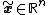is an equilibrium point for the differential equation
Differential equation
A differential equation is a mathematical equation for an unknown function of one or several variables that relates the values of the function itself and its derivatives of various orders...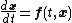if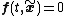for all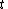.

Similarly, the pointis an equilibrium point (or fixed point
Fixed point (mathematics)
In mathematics, a fixed point of a function is a point that is mapped to itself by the function. A set of fixed points is sometimes called a fixed set...

) for the difference equation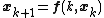if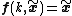for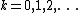.

Equilibria can be classified by looking at the signs of the eigenvalues of the linearization of the equations about the equilibria. That is to say, by evaluating the Jacobian matrix at each of the equilibrium points of the system, and then finding the resulting eigenvalues, the equilibria can be categorized. Then the behavior of the system in the neighborhood of each equilibrium point can be qualitatively determined, (or even quantitatively determined, in some instances, by finding the eigenvector(s) associated with each eigenvalue).

An equilibrium point is hyperbolic if none of the eigenvalues have zero real part. If all eigenvalues have negative real part, the equilibrium is a stable node. If at least one has a positive real part, the equilibrium is an unstable node. If at least one eigenvalue has negative real part and at least one has positive real part, the equilibrium is a saddle point